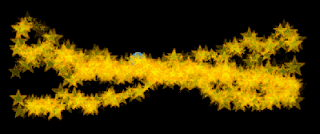It's all about instructions, not memory. Even one memory cell bot can have interesting life if instructions are written properly. To demonstrate this we'll start with most simple bot out there - the crazy bot.

The crazy bot doesn't have any memory. Every frame (or any other given time interval) crazy bot randomly choses its next destination. Create new Movie Clip with linkage name 'bot' and type next code on Timeline first frame (stage size is standard 550x400):

``var _bot:bot = new bot();addChild(_bot);_bot.x = 275;_bot.y = 200;var dx;var dy;addEventListener(Event.ENTER_FRAME, onframe);function onframe(evt:Event):void{    mover(_bot);}function mover(a:bot):void{    dx = Math.random()*10-5;    dy = Math.random()*10-5;    a.x += dx;    a.y += dy;}``

Now, this is one crazy bot! First 4 lines we put our bot in center of the Stage. Next we define dx and dy variables to store frame-by-frame movement. We define event listener to move our bot every frame. We do this in mover function, where every frame we get randomly chosen values between -5 and 5. This is how much our bot will move in pixels in two directions, on x and on y axis.

Now, I'll add only one memory cell to our bot. This cell will store first randomly chosen value for x axis direction. Let's see entire code first:

``var _bot:bot = new bot();addChild(_bot);_bot.x = 275;_bot.y = 200;var info:Object = new Object();info.startX = _bot.x;info.startY = _bot.y;info.memory = new Array();var dx;var dy;function mover(a:bot):void{    if (info.memory.length > 0)     {        if (info.memory > 0)         {            dy = Math.random()*20-10;            dx = Math.random()*10;        } else {            dy = Math.random()*20-10;            dx = Math.random()*(-10);        }    } else {        dy = Math.random()*10-5;        dx = Math.random()*10-5;    }    info.memory = dx;    a.x += dx;    a.y += dy;    info.startX = a.x;    info.startY = a.y;        if( (a.x > 550)||(a.x < 0)||(a.y > 400)||(a.y < 0) )    {        a.x = 275;        a.y = 200;        info.memory = -1 * info.memory;    }    //    var _dot:dot = new dot();//    _dot.x = a.x;//    _dot.y = a.y;//    _dot.scaleX = _dot.scaleY = 1+Math.random()*3;//    _dot.alpha = Math.random()*0.5;//    addChild(_dot);}addEventListener(Event.ENTER_FRAME, onframe);function onframe(evt:Event):void{    mover(_bot);}``

First 4 lines are the same. Next we have info object to store 3 things, starting position of our bot and memory Array. Even though our memory is an Array we will use only first array element, thus our memory has only one cell.

So, inside mover we have 2 if conditional loops, where second one only returns our bot to center of the Stage if it goes out of boundaries. First if loop are instructions. First we check if bot has already moved. If it isn't then memory length is zero and we go to else branch where we choose first move.

Now, if memory cell of our bot does have some value and if that value is positive that means our bot has moved to the right in last frame. We want our bot to continue to move in same direction until it reaches Stage border. That's why we give dx only positive value. On the other hand if our memory cell have negative value, we set dx to some new negative value, so our bot can continue until it reaches left Stage border.

In other words we tell our bot to keep moving in one direction until border, then to return to center and continue in opposite direction and so on ... All this with only 1 memory cell. You can modify dy value if you want him to go more up or down.

Finally, dot MovieClip is random graphic to track bot movement and make interesting drawings. Here are some examples I've made.Now, this is created using only one memory cell. Think of what you can do by using 10 of them or 100?

*_*

1.2.